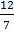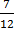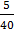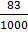### Sample Problem

Write the following ratios as fractions. (You don’t need to simplify)

(a) 12:7

(b) 7 to 12

(c) 5 cm : 40 cm

(d) 83 g to 1 kg

#### Solution

If the units of the two numbers of a ratio are not the same, we need to unify them.

(a) 12:7 =(b) 7:12 =(c) 5 cm : 40 cm =(d) 1 kg = 1000 g, then

83 g to 1 kg = 83 g to 1000 g =